# How to Create a Dynamic Pie Chart in Excel?

In Excel, Pie-chart is a graphical representation of different sections or sectors of a circle based on the proportion, it holds from the complete quantity. Pie-charts are generally categorized into two types:

1. Static Pie-chart: A pie-chart created with static or fixed input values is known to be a static pie-chart. The values of these types of pie-charts don’t change over time.
2. Dynamic Pie-chart: A pie-chart created with dynamic or changing input values is known to be a dynamic pie-chart. The values of these type of pie-charts changes over time.

In this article, we will discuss how to create a dynamic excel pie chart? Creating a dynamic pie chart in Excel means creating a pie-chart that automatically(dynamically) gets updated when new values are entered into the table, which is used for creating the pie-chart. Creating a Dynamic Excel Pie Chart in Excel is very easy. We can create a dynamic pie chart using two different methods:

• Using offset formula
• Using Table

### Creating a dynamic pie chart using offset formula

We can create a dynamic pie chart using the offset formula. So to this follow the following steps:

Step 1: Create a table and insert values in it. Apply the offset formula to get the value of the total column. Here 2000 value is present in the B4 cell. So, to get the first value of the total column, we will have to look for the value in the 0th row and 3rd column from the point of reference. The total column is calculated from the sum of Sale and Tax, so whenever there is any change in the values of the table, the Total column automatically gets updated.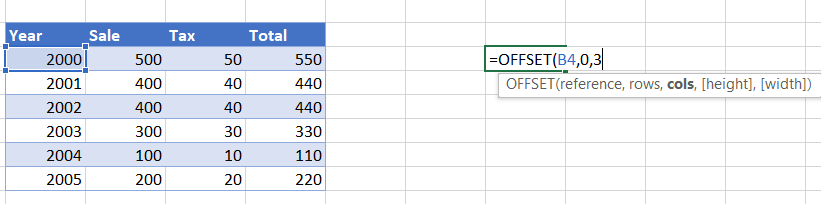Step 2: Total value of 550 appears in the cell.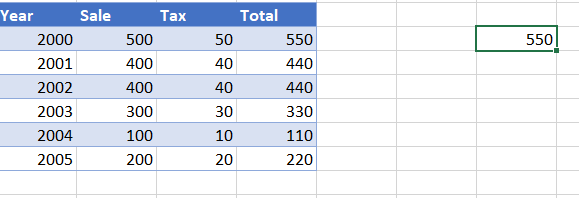Step 3: Now, drag the populated formula to get all the rows of the total column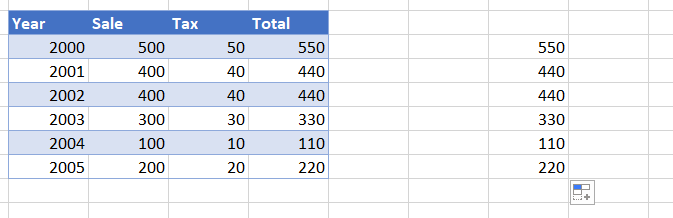Step 4: Select the values that need to be used for creating a dynamic pie-chart. Here, we are using the values calculated from the Offset formula. Click on Insert Menu and select the appropriate pie-chart.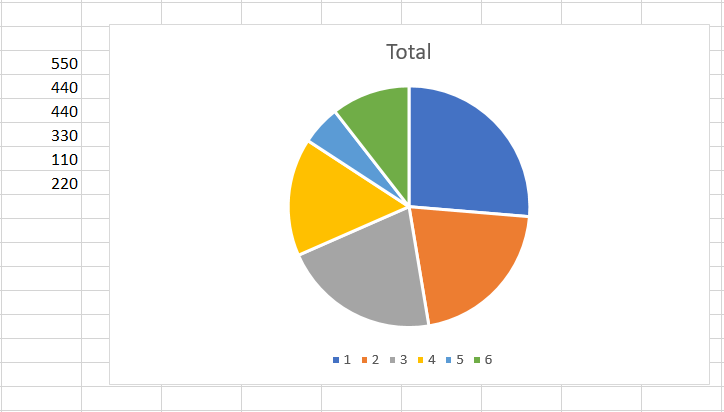Pie-chart gets inserted for the selected values.

### Creating a dynamic pie chart using tables

We can also create a dynamic pie chart using tables. It is the easiest method for creating a dynamic pie chart. So to this follow the following steps:

Step 1: Create a table with proper headings and values inserted in it. Here, a table is created with Year-wise Sale, Tax, and Total(Sum of Sale and Tax) columns.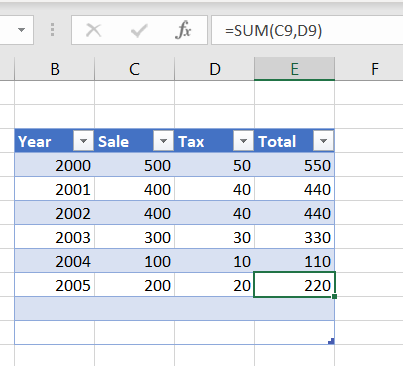Step 2: Copy the headings and paste them separately. Here, Year, Sale, Tax, and Total are pasted separately.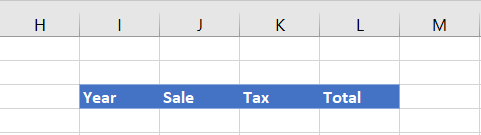Step 3: Select the column with the independent column(Year in the below table). Use Index Function and Counta function to bring the desired value for Year column.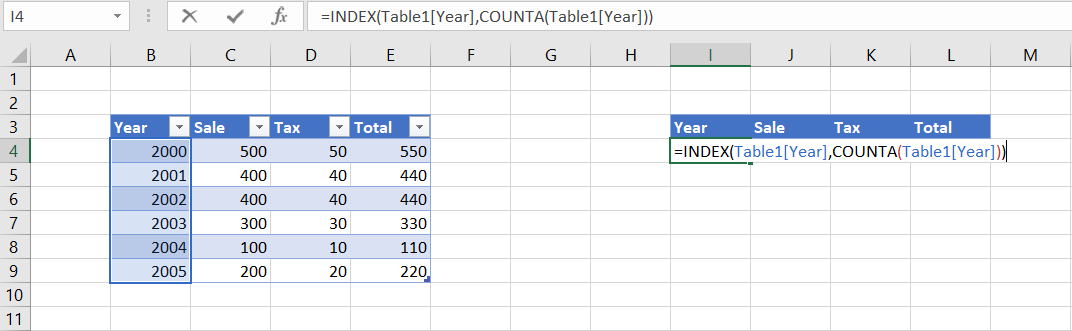Use of index function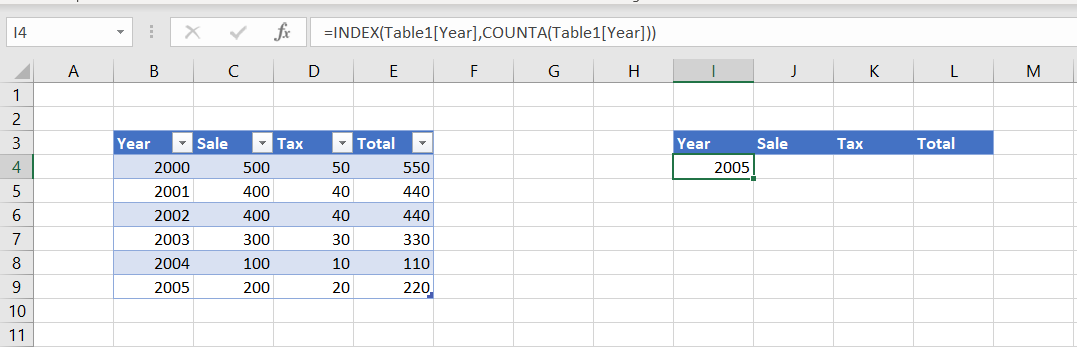Year 2005 is obtained as value for Year

Step 4: Use the Index and Count function for the dependent columns (Sale, Tax) to get their desired value for both the Sale and Tax column.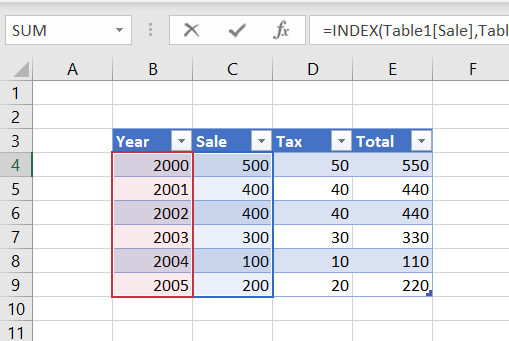Table with selections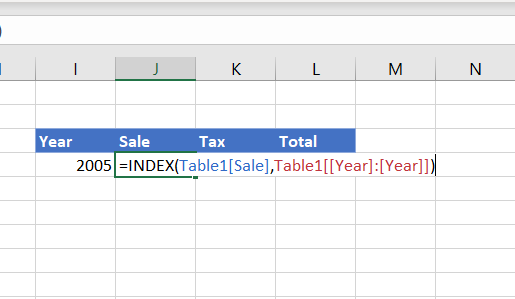Use of Index function

Step 5: Select the columns Sale, Tax, Total. Choose option Pie-chart from Insert menu. A dynamic pie-chart of the table will be created.Year 2005 values in the table are picked for the pie-chart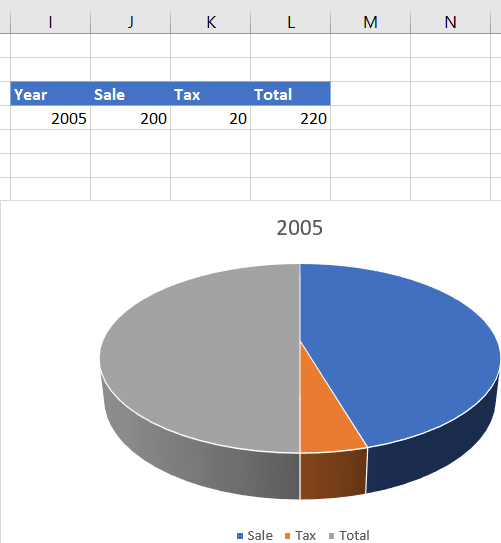Pie-chart for Year 2005

Step 6: Insert a new row in the table. The pie-chart automatically gets updated, as per the new row being added in the table. Here, the pie-chart gets updated for the row with the Year 2006.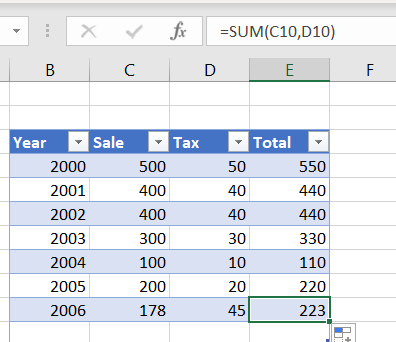Year 2006 values added in the table are picked for the pie-chart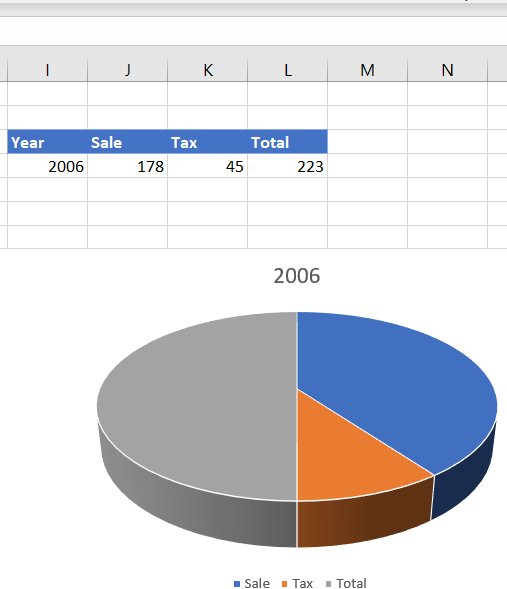Pie-chart for Year 2006

Whether you're preparing for your first job interview or aiming to upskill in this ever-evolving tech landscape, GeeksforGeeks Courses are your key to success. We provide top-quality content at affordable prices, all geared towards accelerating your growth in a time-bound manner. Join the millions we've already empowered, and we're here to do the same for you. Don't miss out - check it out now!

Previous
Next